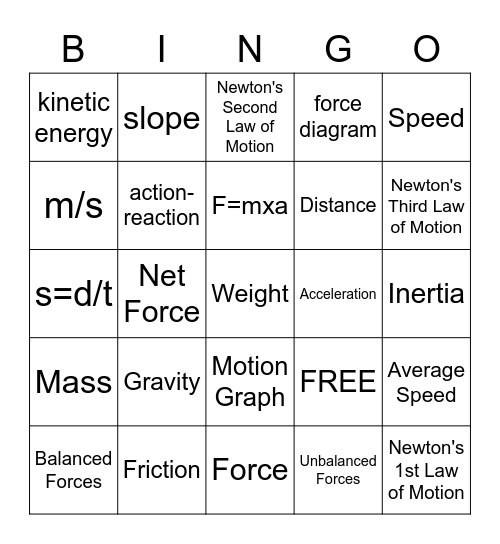# 8th Motion and ForcesThis bingo card has 25 words: Gravity, Force, F=mxa, Balanced Forces, Speed, kinetic energy, m/s, slope, Acceleration, Motion Graph, Distance, s=d/t, FREE, Average Speed, action- reaction, Newton's 1st Law of Motion, Inertia, Newton's Second Law of Motion, Newton's Third Law of Motion, Net Force, Weight, Friction, Mass, force diagram and Unbalanced Forces.

## Play Online